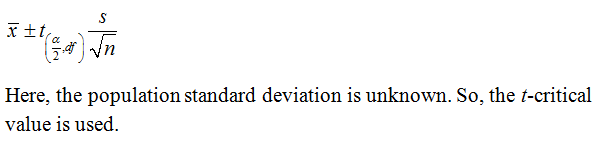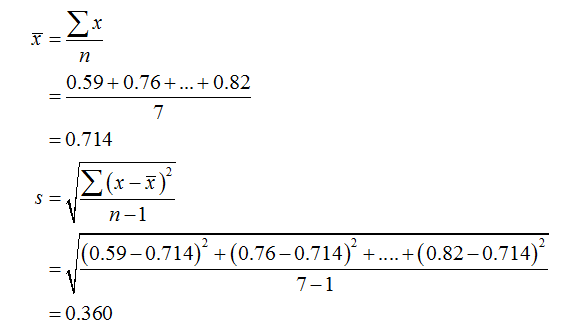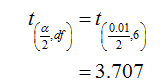# A food safety guideline is that the mercury in fish should be below 1 part per million​ (ppm). Listed below are the amounts of mercury​ (ppm) found in tuna sushi sampled at different stores in a major city. Construct a 99​% confidence interval estimate of the mean amount of mercury in the population. Does it appear that there is too much mercury in tuna​ sushi? 0.59  0.76  0.11  0.92  1.27  0.53  0.82 What is the confidence interval estimate of the population mean μ​?

Question
4 views
A food safety guideline is that the mercury in fish should be below 1 part per million​ (ppm). Listed below are the amounts of mercury​ (ppm) found in tuna sushi sampled at different stores in a major city. Construct a 99​% confidence interval estimate of the mean amount of mercury in the population. Does it appear that there is too much mercury in tuna​ sushi?

0.59
0.76
0.11
0.92
1.27
0.53
0.82

What is the confidence interval estimate of the population mean μ​?
check_circle

Step 1

The provided data is:

0.59,0.76,0.11,0.92,1.27,0.53,0.82

Sample size (n) = 7

The formula to calculate the 99% confidence interval for population mean is:Step 2

The sample mean and sample standard deviation can be calculated as:Step 3

The degrees of freedom is : df= n - 1 = 7 - 1 = 6

The critical value for 99% confidence level at df = 6 can be found using the t-table. That is,...

### Want to see the full answer?

See Solution

#### Want to see this answer and more?

Solutions are written by subject experts who are available 24/7. Questions are typically answered within 1 hour.*

See Solution
*Response times may vary by subject and question.
Tagged in